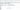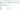# Delete Excel Columns using PowerShell

## Problem#

In this blog post, we will show you how to delete Excel columns using PowerShell.

As the context, our original Worksheet will contain columns as follows:And we will delete all the columns ending with `Name` so that it will look as follows:## Using Excel Com Object#

Since PowerShell is deeply integrated with .NET Framework, we can use `ComObject` to work with Excel files. After creating the object, we will open the Excel file and then remove the columns.

To remove the columns, we must start the iteration from the last index to the first one because when we delete a column, the column on the right will be shifted to the left. This will ensure that we can delete a column while iterating.

``````
try {
# Open excel file
\$excel = New-Object -ComObject Excel.Application
\$workbook = \$excel.Workbooks.Open("C:\Scripts\Test.xlsx")

# Get the first worksheet and modify the headers at A1 and B1
\$worksheet = \$workbook.Worksheets.Item(1)
\$totalColumns = \$worksheet.UsedRange.Columns.Count

for (\$col = \$totalColumns; \$col -ge 1; \$col--) {
if (\$suffix -ieq 'Name') {
\$worksheet.Columns(\$col).Delete()
# Below will also work
# \$worksheet.Cells.Item(1, \$col).EntireColumn.Delete()
}
}

# # Below is the alternative solution if the columns are static
# \$worksheet.Columns(2).Delete()
# \$worksheet.Columns(1).Delete()

# Save excel file
\$workbook.Save()
}
finally {
# Close excel file
\$workbook.Close()

\$excel.Quit()
[System.Runtime.Interopservices.Marshal]::ReleaseComObject(\$excel)
}

``````

We enclose the code above with `try-finally` block because we want to make sure it will release the resource after modifying Excel that is performed at `finally` block.

## Using ImportExcel Module#

`ImportExcel` is an external module that is built based on EPPlus which is a very well-known library to work with Excel spreadsheets in .NET.

Before using `ImportExcel` module, we have to install it.

``````
Install-Module -Name ImportExcel

``````

And then following previous solution pattern, we have to open the file first before removing the columns.

``````
try {
# Import the module
Import-Module ImportExcel

# Open excel file
\$excel = Open-ExcelPackage -Path "C:\Scripts\Test.xlsx"

# Get the first worksheet and modify the headers at A1 and B1
\$workSheet = \$excel.Workbook.Worksheets
\$startColumn = \$workSheet.Dimension.Start.Column
\$endColumn = \$workSheet.Dimension.End.Column

for (\$col = \$endColumn; \$col -ge \$startColumn; \$col--) {
if (\$suffix -ieq 'Name') {
\$worksheet.DeleteColumn(\$col)
}
}

# # Below is the alternative solution if the columns are static
# \$worksheet.DeleteColumn(2)
# \$worksheet.DeleteColumn(1)
}
finally {
# Close excel file
Close-ExcelPackage \$excel
}

``````

Similar to previous solution, we enclose the script with `try-finally` block to avoid memory leak.

## Using PSExcel Module#

`PSExcel` is another module based on EPPlus library. You can find all the examples in github repository.

Before using this module, we have to install it.

``````
Install-Module -Name PSExcel

``````

In order to use the object, we must import the module first. Then, we create Excel object by specifying the path of our Excel file. This object will be used to get the first worksheet whose columns will be removed.

``````
try {
# Import the module
Import-Module PSExcel

# Open excel file
\$excel = New-Excel -Path 'C:\Scripts\Test.xlsx'

# Get the first worksheet and modify the headers at A1 and B1
\$workSheet = \$excel.Workbook.Worksheets
\$startColumn = \$workSheet.Dimension.Start.Column
\$endColumn = \$workSheet.Dimension.End.Column

for (\$col = \$endColumn; \$col -ge \$startColumn; \$col--) {
if (\$suffix -ieq 'Name') {
\$worksheet.DeleteColumn(\$col)
}
}

# # Below is the alternative solution if the columns are static
# \$worksheet.DeleteColumn(2)
# \$worksheet.DeleteColumn(1)

# Save excel file
\$excel | Save-Excel
}
finally {
\$excel | Close-Excel
}

``````

We also enclose the script with `try-finally` block to avoid memory leak.

## Conclusion#

To remove Excel columns using PowerShell, we can use `Excel Com Object` which is based on .NET Framework. We can also use PowerShell external modules like `PSExcel` and `ImportExcel`. `ImportExcel` is an excellent module which is based on `EPPlus` library, a well-known C# libary for woking with Excel from .NET.

`PSExcel` is the alternative of `ImportExcel` module. `PSExcel` is also based on `EPPlus` but this module is no longer maintained as stated in its GitHub repository. If it doesn’t provide functionality that you need, you have to contribute to the source code by yourself.